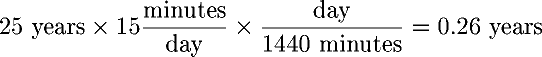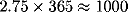Next: Calculating feedback models Up: Pencil and Paper Previous: Forecasting weight loss

## Forecasting life extension

To calculate the extra minutes per day equivalent to extending your life by a given number of years, start with a guess at your minimum lifespan, overweight and out of shape. If you're less than 60, use 65 years, figuring on dropping dead on the day you retire. Life's like that, isn't it? If you're 60 or above, add 10 years to your current age. Next, subtract your age from this number, giving the years you'd live in your present shape. For example, if you're 40 now, and assuming you'd live to 65 without losing weight or exercising, you'd calculate 65-40=25 years to live.

Next, make an assumption about the number of years you'll increase your life expectancy by exercising. The figure of 3 years used in chapteris conservative; some estimates run as high as 4 years reduced expectancy simply from being 15 pounds overweight. Multiply the years of increased lifespan by 1440, the number of minutes in a day (24×60=1440), to obtain the total minutes of increased lifespan, then divide by the number of years you'd live otherwise to obtain the extra minutes per day. Assuming 3 years of increased life span and 25 years to live otherwise, you'd calculate:Thus, if losing weight and exercising extends your life by 3 years past age 65, you've added extra time to your life equivalent to more than 172 minutes a day: almost three extra hours!

But at what cost in time? If you extend your life 3 years past age 65 with 15 minutes of daily exercise, you have a net gain of 172-15=157 minutes a day, still more than two and a half hours. Another perspective on the time invested in a longer life is given by calculating the total years you'll spend exercising from now until the end of your estimated lifespan. Take your estimated years to live, multiply by the minutes per day spent exercising, and divide by 1440. Using the same assumptions as before, and figuring on 15 minutes a day of exercise, yields:Thus, in the 25 years from age 40 to 65, you'd spend a total of about a quarter of a year exercising. If that bought you three more years to live, you'd end up with 2 3/4 extra years, more than 1000 additional days (), to enjoy life, even if you figure the time spent on exercise was otherwise completely wasted and you derived no other benefits from it apart from a longer life span.Next: Calculating feedback models Up: Pencil and Paper Previous: Forecasting weight loss

By John Walker